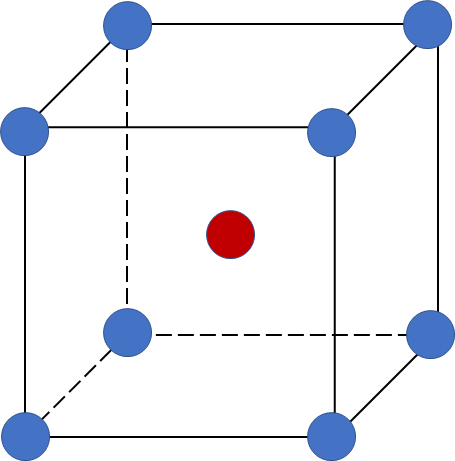# Problem: Tantalum (Ta; d = 16.634 g/cm3 and ℳ = 180.9479 g/mol) has a body-centered cubic structure with a unit-cell edge length of 3.3058 Å. Use these data to calculate Avogadro’s number.

###### FREE Expert Solution

A body-centered cubic (BCC) unit cell is composed of a cube with one atom at each of its corners and one atom at the center of the cubeRecall the # of atoms present per 1 BCC unit cell: corner atoms contribute 1/8 and the center atom contribute 1

# of atoms = 2 per 1 unit cell

To calculate Avogadro's number: (# of entities in 1 mole)

Step 1: Volume of unit cell

volume of unit cell = 3.61268 x 10-23 cm3

97% (349 ratings)###### Problem Details

Tantalum (Ta; d = 16.634 g/cm3 and ℳ = 180.9479 g/mol) has a body-centered cubic structure with a unit-cell edge length of 3.3058 Å. Use these data to calculate Avogadro’s number.